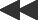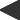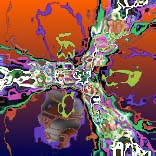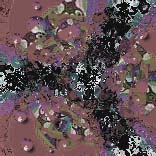basis: newton-fractal Based on the Newton formula for finding the roots of zp - 1. Pixels are colored according to the iteration when the orbit is captured by a root. z(0) = pixel; z(n+1) = ((p-1)*z(n)p + 1) / (p*z(n)(p - 1)) One parameter: the polynomial degree p.The Newton formula, named after Isaac Newton, is an algorithm used to find the roots of polynomial equations by successive "guesses" that converge on the correct value as you feed the results of each approximation back into the formula. It works very well - unless you are unlucky enough to pick a value that is on a line between two actual roots. In that case, the sequence explodes into chaos, with results that diverge more and more wildly as you continue the iteration.